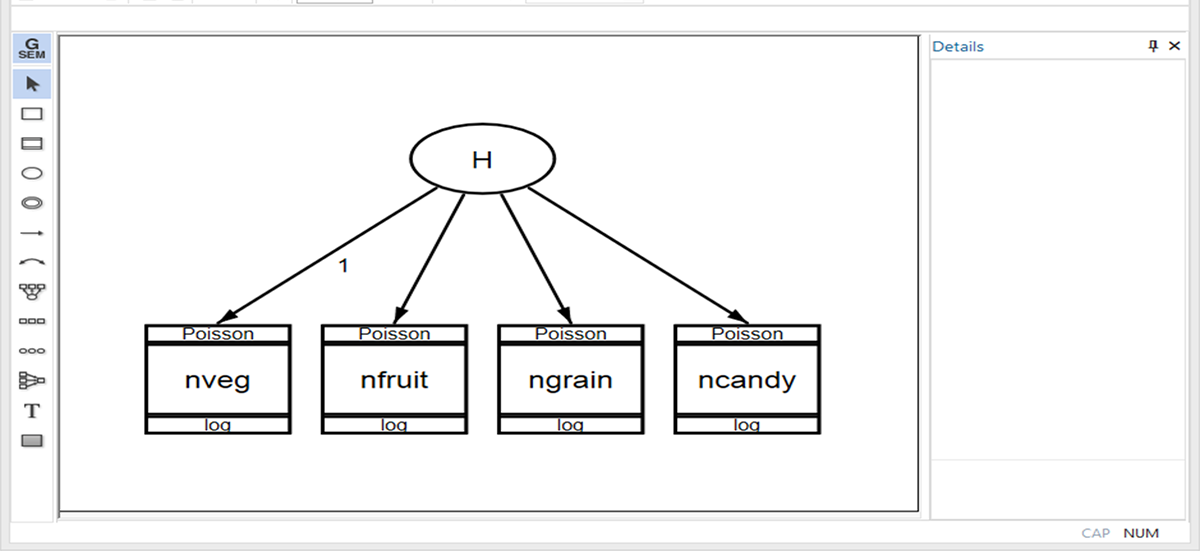»  Home »  Products »  Features »  Multiple-group generalized SEM

## Multiple-group generalized SEM

### Highlights

• Group-specific estimates in
• Multilevel SEM
• SEM with continuous, binary, ordinal count, categorical, and survival outcomes
• Test for group invariance
• Support for complex survey data

Stata's generalized structural equations model (SEM) command makes it easy to fit models on data comprising groups.

With gsem's features, you can perform a confirmatory factor analysis (CFA) and allow for differences between men and women by typing

. gsem (nveg@1 nfruit ngrain ncandy <- H), poisson
group(female)
ginvariant(none)
mean(H@0)


If you are new to Stata and gsem, let us tell you that this is just one feature in a command that already has many features. gsem fits confirmatory factor models, seemingly unrelated models, SEMs, multilevel models, and all combinations thereof. It fits these models with outcomes that are continuous, binary, ordinal, count, and even survival. With the group() option, we can estimate distinct parameters across groups for any of these models. We can even combine group analysis with gsem's latent class analyses feature.

### Syntax features

Syntax features group () and ginvariant() are options. They work together.

Say you want to fit a path model such as

. gsem (y1 <- y2 x1, poisson) (y2 <- x1 x2)


If you wanted to fit the same model but obtain separate parameter estimates for each of three groups in the data identified by variable subset equal to 1, 2, and 3, you could fit the model three times:

. gsem (y1 <- y2 x1, poisson) (y2 <- x1 x2) if subset==1

. gsem (y1 <- y2 x1, poisson) (y2 <- x1 x2) if subset==2

. gsem (y1 <- y2 x1, poisson) (y2 <- x1 x2) if subset==3


But then you could not compare the fitted parameters or constrain some parameters to be equal across groups.

In Stata, you can type

. gsem (y1 <- y2 x1, poisson) (y2 <- x1 x2),
group(subset) ginvariant(none)


And you can specify a separate model for each group:

. gsem (1: y1 <- y2 x1,    poisson) (1: y2 <- x1 x2   )
(2: y1 <- y2 x1 x3, poisson) (2: y2 <- x1 x2   )
(3: y1 <- y2 x1,    poisson) (3: y2 <- x1 x2 x4),
group(subset) ginvariant(none)



The ginvariant() option specifies which fitted parameters are to be constrained to be equal across groups. The types of parameters gsem fits are



fitted                ginvariant() suboption

intercepts            cons
coefficients          coef
error variances       errvar
scalar parameters     scale
latent means          means
latent covariances    covex

none
all

coefficients.



Thus, if you type

. gsem (y1 <- y2 x1, poisson) (y2 <- x1 x2),
group(subset) ginvariant(cons)


only the intercepts are constrained to be equal across groups.

### Let's see it work

We have simulated data from a nutrition study where people kept a food diary for two weeks. In this diary, each person tallied the number of servings of vegetables, fruits, grains, and candy they consumed that day. The data contain the two-week totals for each person in the study and a variable indicating whether the person was male or female.

We want to perform a CFA. The serving totals are believed to represent measures of a latent trait, H, which we will call healthy eating inclination. We will anchor the latent trait to the total for vegetables.

Initially, we might fit a CFA model without accounting for the participant's sex by typing

. gsem (nveg@1 nfruit ngrain ncandy <- H), poisson


or by drawing the path diagram in the BuilderHowever, we imagine the study was intended to determine the differences between the male and female participants. So instead, we type

. gsem (nveg@1 nfruit ngrain ncandy <- H), poisson
group(female)
ginvariant(none)
mean(H@0)


We add option group(female) to fit the model separately for males and females.

We add option ginvariant(none) to allow all parameters to vary between males and females.

We add option mean(H@0) because we assume the latent trait is centered at zero for both groups. (It also makes this model identified because H is a latent variable and each group has its own intercepts.)

To fit the multiple-group model from the Builder, we draw the same path diagram that we drew without groups. When we are ready to fit the model, we select the equivalent of the command options from the dialog box.

Whether we used the command or the Builder, we have now fit the CFA model that allows distinct intercepts, coefficients, and variances of the latent variable across groups.

The output with all estimates for the two groups is a bit lengthy, so we will not show it here. But we will tell you that the estimates of the coefficients, the intercepts, and the variances are similar for the two groups.

Say we want to test for parameter invariance—whether the parameters are equal for males and females. We could perform this test for an individual parameter, for a group of parameters such as all coefficients, or for all parameters. We could use a Wald test or a likelihood-ratio test.

If we wanted to do a Wald test, we would use Stata's test command. There is nothing new here.

If we wanted to do a likelihood-ratio test comparing the model with all parameters constrained and the model with all parameters estimated distinctly for males and females, we could refit the model with ginvariant(all) and use lrtest:

. estimates store unconstrained

. gsem (nveg@1 nfruit ngrain ncandy <- H), poisson
group(female)
ginvariant(all)
mean(H@0)
. estimates store constrained

. lrtest unconstrained constrained


In our case, the results are

. lrtest unconstrained constrained

Likelihood-ratio test                                 LR chi2(8)  =      4.98
(Assumption: constrained nested in unconstrained)     Prob > chi2 =    0.7595

We find no evidence that the model with distinct parameters fits better than the model with all parameters constrained. Measurement of healthy eating inclination does not appear to differ for men and women.